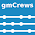### The Guided Cantilever Method - Part 3

The Guided Cantilever Method for Quickly Checking Piping Flexibility -- Part 3

This is a continuation of my last post. Here in Part 3, I show how to manually check more complicated piping systems for adequate thermal flexibility. There are two tricks for doing this: 1) fictitious anchors and 2) the-tail-does-not-wag-the-dog.

Fictitious Anchors

The idea here is that any point or points on a complicated piping network can be fixed against rotation and/or displacement (or a fixed displacement or rotation can be imposed at any point) and the flexibility of the resulting subsystems analyzed independently. (The fixed points isolate one part of the system from the others.) The importance of this is that if each subsystem has adequate flexibility with fixed points in place, then the overall system will have adequate flexibility with the fixed points removed. (Equal sized pipe only. For unequal pipe sizes, see next trick.)

An example should make this clear:
Notice that for analysis purposes, an imaginary fictitious anchor has been added to the system illustrated in Figure 2. This allows us to use the guided cantilever method since the system has been broken up into two simple subsystems similar to what we solved for last time in Part 2. There is a 20'X20' subsystem and a 10'X10' subsystem.

Note that the required length of a guided cantilever goes up with the square root of the displacement to be absorbed. This means that if the 10'X10' subsystem is adequately flexible then the 20'X20' subsystem will be even more adequately flexible. And so if the 10'X10' subsystem is OK, then the entire system will be OK when the imaginary anchor is "removed".

So is the 10'X10' subsystem adequately flexible?
``````from math import sqrt

# The thermal displacement to be absorbed (y) is:
#     Material = stainless steel
#     Length = 10 (ft)
#     Temperature = 350 (F)
#     Formula from last post:
#         y_per_100ft = 1.33 * (T - 100.) / 100.
y_per_100ft = 1.33 * (350. - 100.) / 100.
y = y_per_100ft * (10 / 100)
# y = .3325 (in)

# So that the required leg length to absorb this expansion is:
NPS = 4
L = 7.77 * sqrt(y * NPS)
# L = 9.0 (ft)
``````
Thus, the 10'X10' subsystem is adequately flexible. Thus, the entire system is adequately flexible.

Although it takes some practice to using fictitious anchors effectively, once you've gotten the hang of it, I have found fictitious anchors are a very effective tool.

The Tail Does Not Wag the Dog

Recall that resistance to bending moment is proportional to section modulus. And that the section modulus of pipe is proportional to its radius to the third power. Thus, all other things being proportional, larger diameter piping will dominate the thermal displacement reactions of complex piping systems versus smaller diameter pipe. For example, the section modulus of a 6" NPS sch. 40 pipe is 8.50 (in3) while the section modulus of a 3" NPS sch. 40 pipe is 1.72 (in3). A strength ratio of almost 5 to 1, even though the size ratio is less than 2 to 1. Thus, a 3" NPS "tail" will not wag a 6" NPS "dog".

This means that in complicated piping systems with varying pipe sizes, it is appropriate for the fictitious anchors to be placed and to impose movements that would otherwise be the unrestrained displacements of the larger pipe sizes.

For example, in Figure 2 above, if the 20'X20' subsystem were 8" NPS pipe instead of 4", and there were an 8X4 reducer at the fictitious anchor, it would be appropriate to assume the fictitious anchor would impose 20' worth of thermal expansion on the 10'X10' subsystem instead of being fixed in place. Clearly, the 10'X10' subsystem would then fail a manual check and the entire system would have to be computer analyzed.

Summary

In practice, it is easily possible to manually analyze for flexibility a majority of piping systems in less time than it would take to perform computer analyses on these systems. All piping engineers and designers should be familiar with the guided cantilever method.

#### 1 comment:

1.This is one of four posts on the guided cantilever method:

Part 1
Part 2
Part 3
Part 4